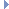﻿ MPlane Class Reference

# MPlane Class Reference

`#include <MPlane.h>`

## Class Description

A plane equation.

This class describes a mathematical plane.Examples:

## Public Member Functions

MPlane ()
Class default constructor. More...

MPlane (const MPlane &)
Class copy constructor. More...

MVector normal () const
Returns the normal of the plane. More...

double distance () const
Returns the distance of the plane along the normal. More...

void setPlane (double a, double b, double c, double d)
Directly set the equation of the plane in the form: ax + by +cz + d = 0. More...

void setPlane (const MVector &n, double d)
Set the equation of the plane from a normal and offset. More...

double distance (const MVector &) const
Returns the unsigned distance from the plane to the specified point. More...

double directedDistance (const MVector &) const
Returns the signed distance from the plane to the specified point. More...

## Static Public Member Functions

static const char * className ()
Returns the name of this class. More...

## Constructor & Destructor Documentation

 MPlane ( )

Class default constructor.

Create a new default plane.

 MPlane ( const MPlane & src )

Class copy constructor.

Create a new vector and initialize it to the same values as the given plane.

Parameters
 [in] src the plane to copy

## Member Function Documentation

 MVector normal ( ) const

Returns the normal of the plane.

Returns
The plane's normalExamples:
 double distance ( ) const

Returns the distance of the plane along the normal.

Returns
The distance of the plane along the normal
 void setPlane ( double a, double b, double c, double d )

Directly set the equation of the plane in the form: ax + by +cz + d = 0.

Parameters
 [in] a The plane equation's x coefficent [in] b The plane equation's y coefficent [in] c The plane equation's z coefficent [in] d The plane equation's constant distance term
 void setPlane ( const MVector & n, double d )

Set the equation of the plane from a normal and offset.

Parameters
 [in] n The plane's normal [in] d The offset of the plane along the normal
 double distance ( const MVector & point ) const

Returns the unsigned distance from the plane to the specified point.

Parameters
 [in] point The point from which to calculate the distance
Returns
The distance from the specified point to the plane.
 double directedDistance ( const MVector & point ) const

Returns the signed distance from the plane to the specified point.

Parameters
 [in] point The point from which to calculate the distance
Returns
The distance from the specified point to the plane.Examples:
 const char * className ( )
static

Returns the name of this class.

Returns
The name of this class.

The documentation for this class was generated from the following files:
• MPlane.h
• MPlane.cpp